﻿ Thermal Analysis of IPM MotorExamples | Product | Murata Software Co., Ltd.# Example1Thermal Analysis of IPM Motor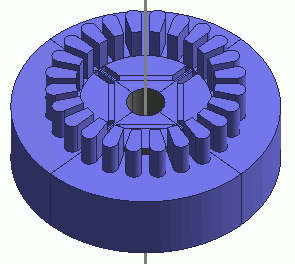General

• Temperature and torque characteristics of IPM motor (Interior Permanent Magnet Motor) are analyzed when the electricity is applied.
The model is a permanent magnet synchronous motor with magnets built in a rotor.

• The analysis is coupled with the external circuit.

• The homogenizing method is applied to simulate the layer structure of the electromagnetic plates of steel for the core.

• Fast stabilizer is employed to make the magnetic field reach the steady state in short calculation time.

• Temperature, torque and magnetic flux density are solved.

• Unless specified in the list below, the default conditions will be applied.

### Analysis Conditions

 Item Setting Solver Magnetic Field Analysis [Luvens] Thermal Analysis [Watt] Analysis Type Magnetic field analysis: Transient Analysis Thermal analysis: Steady-State Analysis Unit mm Options Select External Circuit Coupling. Select Rotating machinery.   [Conversion] Model Thickness: 30×10^-3[m] Select Partial Model. Number of Divisions of the Whole Model: 4 Circuit Configuration Number of Series: 1 Circuit Configuration Number of parallels: 1 Select Convert the result to the whole model and output it.

The Rotating Machinery tab is set as follows.

 Tab Setting Item Setting Rotating Machinery Rotational Movement Select Constant Velocity. Number of Rotations: 1800[r/min] Rotor’s Initial Rotation Position: 0[deg] Number of Slide Mesh Divisions Circumferential Division Angle: 1.0[deg] Rotation per Step: 1[mesh] Number of Slide Mesh Layers: 3

External circuit is as follows.

60[Hz] three-phase AC voltage is applied.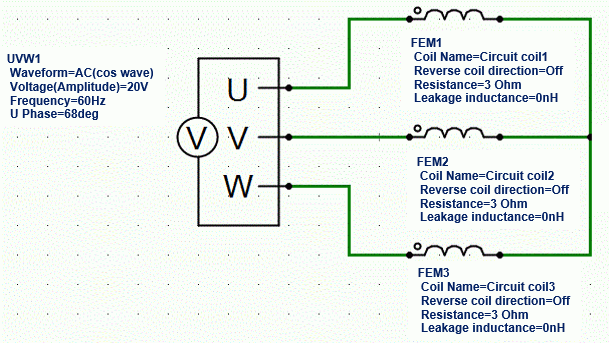Set the Mesh Tab as follows.

 Tab Setting Item Setting Mesh Meshing Setup Automatically set the general mesh size: Deselect General mesh size: 4[mm] Ambient Air Creation Select Create ambient air automatically. Ambient Air Scale: 1.2

The Transient Analysis tab is set up as follows.

With the settings as below, number of steps is 450, circumferential division angle is 1.0[deg], and rotation per step is 1[mesh], the rotation up to 450 degrees (=450*1.0*1) is analyzed.

The steps are set until the magnetic field reaches the steady state.

Tab

Setting Item

Setting

Transient Analysis

Time step

Automatic

Table

 Number Calculation steps Output steps 1 450 1

Fast stabilizer is set up as follows to make the magnetic field reach the steady state in short calculation time.

 Tab Setting Item Setting Fast Stabilizer Correction Method Simplified Three-Phase AC TP-EEC Method Coil Name on the External Circuit U Coil: Circuit_Coil1 V Coil: Circuit_Coil2 W Coil: Circuit_Coil3

### Graphical Objects

A rotor core and magnet are placed in the center. A stator and coils are placed around them.

This is a 3D model analysis. By utilizing the symmetry of the model, it is made to be a quarter model.

Half period rotation boundary (symmetry) is set.

“Ambient Air Creation” is selected.

Adiabatic boundary condition is set on the shaft and the gap between stator and rotor.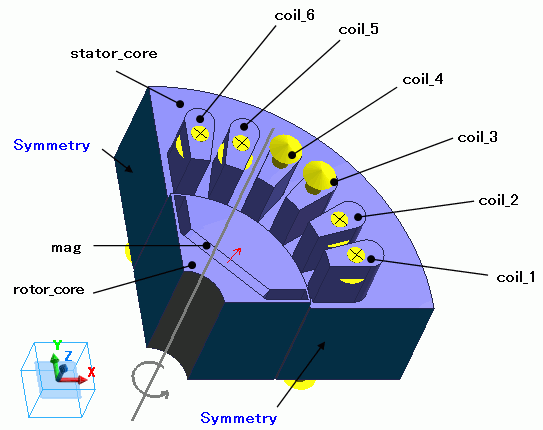### Body Attributes and Materials

 Body Number/Type Body Attribute Name Material Name 28/Solid coil_1 Cu 34/Solid coil_2 Cu 35/Solid coil_3 Cu 36/Solid coil_4 Cu 37/Solid coil_5 Cu 38/Solid coil_6 Cu 55/Solid mag mag 45/Solid stator_core core 47/Solid rotor_core core

The body attribute is set up as follows.

For the core, the homogenizing method is selected to simulate the layered steel plates.

 Body Attribute Name Tab Setting coil_1 Current Waveform: External Circuit Coupling Coil Name on the Circuit: Circuit_Coil1 Turns: 35[Turns] Direction: Specify Inflow/Outflow Faces Stator/Rotor/Air Stator coil_2 Current Waveform: External Circuit Coupling Coil Name on the Circuit: Circuit_Coil1 Turns: 35[Turns] Direction: Specify Inflow/Outflow Faces Stator/Rotor/Air Stator coil_3 Current Waveform: External Circuit Coupling Coil Name on the Circuit: Circuit_Coil2 Turns: 35[Turns] Direction: Specify Inflow/Outflow Faces Stator/Rotor/Air Stator coil_4 Current Waveform: External Circuit Coupling Coil Name on the Circuit: Circuit_Coil2 Turns: 35[Turns] Direction: Specify Inflow/Outflow Faces Stator/Rotor/Air Stator coil_l5 Current Waveform: External Circuit Coupling Coil Name on the Circuit: Circuit_Coil3 Turns: 35[Turns] Direction: Specify Inflow/Outflow Faces Stator/Rotor/Air Stator coil_6 Current Waveform: External Circuit Coupling Coil Name on the Circuit: Circuit_Coil3 Turns: 35[Turns] Direction: Specify Inflow/Outflow Faces Stator/Rotor/Air Stator mag Direction Vector: X=1, Y=1, Z=0 Stator/Rotor/Air Rotor rotor_core Layer Select Take layer into account Space: 97[%] Layer Direction Vector: X=0, Y=0, Z=1 Stator/Rotor/Air Rotor stator_core Layer Select Take layer into account Space: 97[%] Layer Direction Vector: X=0, Y=0, Z=1 Stator/Rotor/Air Stator

The material properties are set as follows.

Material Name

Tab

Properties

Cu

Conductivity

Conductivity Type: Conductor

Conductivity: 5.977×10^7[S/m]

Thermal Conductivity

Thermal Conductivity: 398[W/m/deg]

mag

Relative permeability

Material Type: Permanent Magnet

Magnet

Magnetization Characteristic Type: Linear

Magnetization Strength: 1.25

Relative Permeability: 1.05

Thermal Conductivity

Thermal Conductivity: 1[W/m/deg]

core

Electric Conductivity

Conductivity Type: Conductor

Conductivity: 1.7 x10^6[S/m]

Permeability

Magnetization Characteristic Type: Select B-H curve

B-H Curve Table

 Magnetic Field [A/m] Magnetic Flux Density [T] 0 0 58 0.42 90 0.8 180 1.19 380 1.37 1100 1.48 2000 1.55 3000 1.608 11000 1.81 20000 1.91

Thermal Conductivity

Thermal Conductivity: 10[W/m/deg]

### Boundary Conditions

“Natural convection (automatic calculation)” is set on the outer boundary condition (surrounding the motor in the case of the thermal analysis).

Half period symmetric boundary is set.

Adiabatic boundary condition is set on the shaft and the gap between stator and rotor.

 Boundary Condition Name/Topology Tab Boundary Condition Type Setting Outer Boundary Condition * Thermal Heat Transfer/Ambient Radiation Natural convection (automatic calculation) Room temperature: 25[deg] shaft Thermal Adiabatic rotor Thermal Adiabatic stator Thermal Adiabatic Symmetry Symmetry/Continuity Periodic Rotation Period (Half Period)

To set Outer Boundary Condition, go to the [Model] tab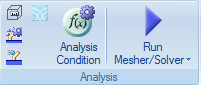and click [Outer Boundary Condition]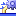.

### Results

The distribution of the magnetic flux density at 450 calculation steps.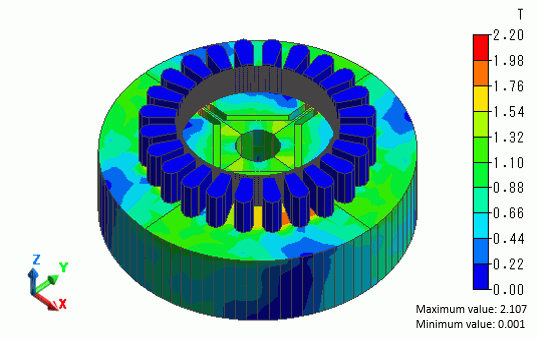The diagram below shows time-torque characteristics.

Transient state becomes stable at around 20[ms].

About 0.5[N*m] of torque is obtained.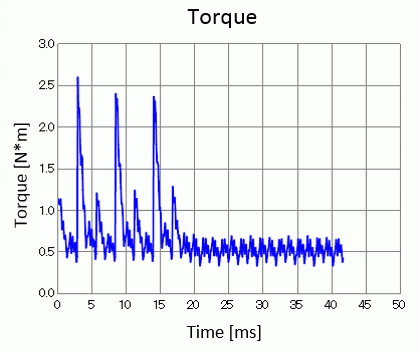The diagram below shows the temperature distribution of the stator.

In the steady state, it is around 100[deg].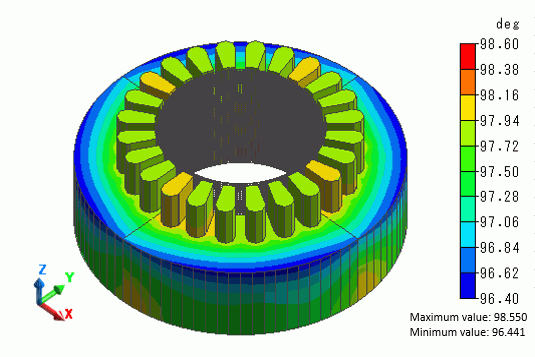﻿